# Beam deflection

## Homework Statement

determine deflection of beam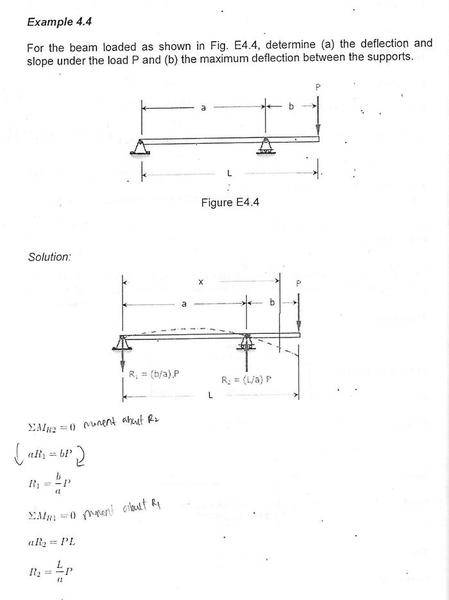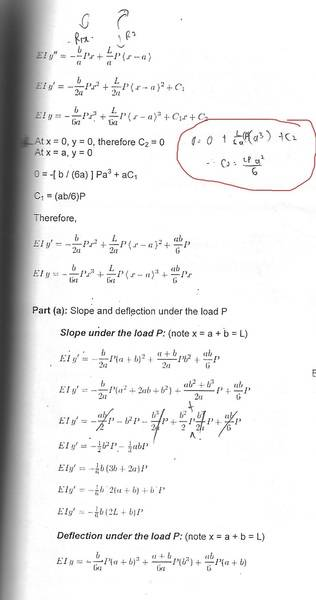## The Attempt at a Solution

at x=0, y=0 , i found that C2 = LP(a^2)/6 (circled part) but the author gt 0, which is correct?

SteamKing
Staff Emeritus
Homework Helper

## Homework Statement

determine deflection of beam## The Attempt at a Solution

at x=0, y=0 , i found that C2 = LP(a^2)/6 (circled part) but the author gt 0, which is correct?

The author is correct.

##EIy = -\frac{b}{6a}Px^3 + \frac{L}{6a}P<x-a>^3+C_1x+C_2##

When x = 0, y = 0, and the expression <x-a> = 0, due to the definition of the singularity function <x-a>, and C2 = 0 as described in the text.

The author is correct.

##EIy = -\frac{b}{6a}Px^3 + \frac{L}{6a}P<x-a>^3+C_1x+C_2##

When x = 0, y = 0, and the expression <x-a> = 0, due to the definition of the singularity function <x-a>, and C2 = 0 as described in the text.
why <x-a> = 0 ?

SteamKing
Staff Emeritus
Homework Helper
why <x-a> = 0 ?
The way a singularity function is defined:

<x-a> = 0, if x - a < 0
<x-a> = 1, if x - a > 0

https://en.wikipedia.org/wiki/Singularity_function

You should check your text for the precise definition.

•The way a singularity function is defined:

<x-a> = 0, if x - a < 0
<x-a> = 1, if x - a > 0

https://en.wikipedia.org/wiki/Singularity_function

You should check your text for the precise definition.
my notes doesnt hv explaination on this

The way a singularity function is defined:

<x-a> = 0, if x - a < 0
<x-a> = 1, if x - a > 0

https://en.wikipedia.org/wiki/Singularity_function

You should check your text for the precise definition.
since x - a > 0, so <x-a> = 1 , am i right ? why the author take <x-a> = 0 ?

SteamKing
Staff Emeritus
Homework Helper
since x - a > 0, so <x-a> = 1 , am i right ? why the author take <x-a> = 0 ?
When x = 0, <0 - a> = -a; -a < 0; <0-a> = 0, according to the definition of the singularity function

SteamKing
Staff Emeritus
Homework Helper
my notes doesnt hv explaination on this
What about your textbook? Do you have a textbook for this course? What does it say?

What about your textbook? Do you have a textbook for this course? What does it say?
ok , i have another part of question here . in the working , the maximum deflection occur at x= (1/sqrt rt 3) ,
why the author ignore the LP[(x-a)^3]/ (6a) at x = (1/sqrt rt 3) ?
by ignoring the at LP[(x-a)^3]/ (6a) at x= (1/sqrt rt 3) , the author assume x-a <0 ????
but , the value of a is unknown , how to know that x-a<0 ?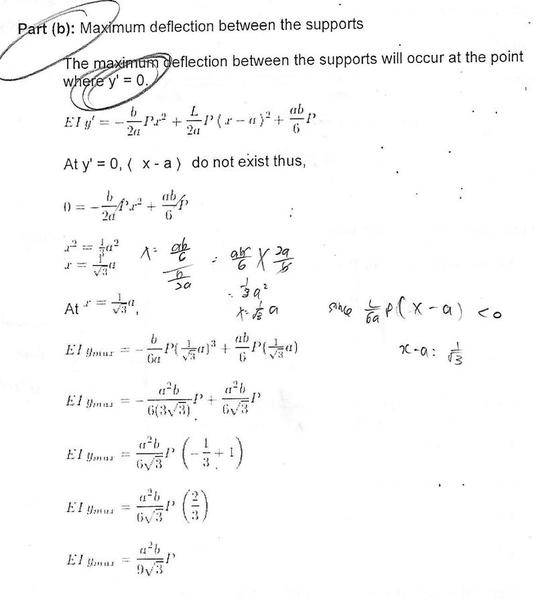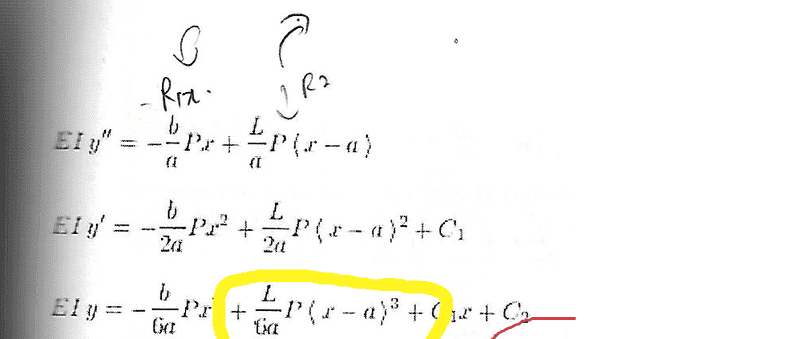Last edited:
SteamKing
Staff Emeritus
Homework Helper
ok , i have another part of question here . in the working , the maximum deflection occur at x= (1/sqrt rt 3) ,
why the author ignore the LP[(x-a)^3]/ (6a) at x = (1/sqrt rt 3) ?
by ignoring the at LP[(x-a)^3]/ (6a) at x= (1/sqrt rt 3) , the author assume x-a <0 ????
but , the value of a is unknown , how to know that x-a<0 ?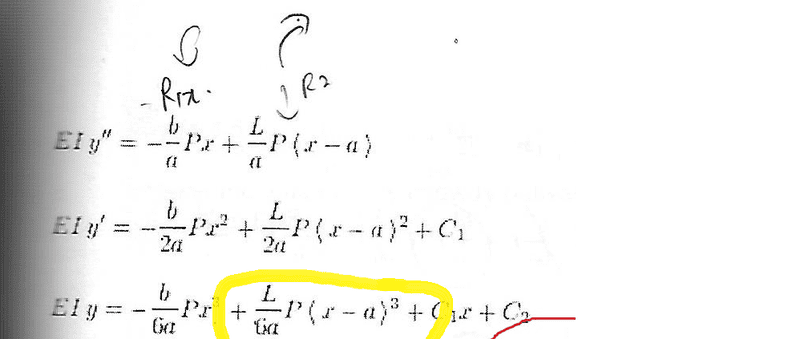I think the author is clumsily trying to say that the singularity function <x-a> = 0 where the deflection is at a maximum. Instead of saying "do not exist", it would probably be better to say that "<x-a> vanishes" at that location.

Anyhow, once that fact is recognized, then the location x of the maximum deflection can be solved for as illustrated.

•I think the author is clumsily trying to say that the singularity function <x-a> = 0 where the deflection is at a maximum. Instead of saying "do not exist", it would probably be better to say that "<x-a> vanishes" at that location.

Anyhow, once that fact is recognized, then the location x of the maximum deflection can be solved for as illustrated.
how could that be ? the value of a is unknown

SteamKing
Staff Emeritus
Homework Helper
how could that be ? the value of a is unknown
No, it isn't. a is the distance between R1 and R2. Look at the beam diagram.

No, it isn't. a is the distance between R1 and R2. Look at the beam diagram.
if it' so , x > a , so x-a > 0 , so <x-a> should be =1 , right ?

SteamKing
Staff Emeritus
Homework Helper
if it' so , x > a , so x-a > 0 , so <x-a> should be =1 , right ?
It's not a which is unknown, it is the value of x at which the deflection is a maximum.

•I think the author is clumsily trying to say that the singularity function <x-a> = 0 where the deflection is at a maximum. Instead of saying "do not exist", it would probably be better to say that "<x-a> vanishes" at that location.

Anyhow, once that fact is recognized, then the location x of the maximum deflection can be solved for as illustrated.
why <x-a> = 0 when deflection is at a maximum ??

SteamKing
Staff Emeritus
Homework Helper
why <x-a> = 0 when deflection is at a maximum ??
Specifically, the problem is looking for the location of the maximum deflection between the supports.

The quantity <x-a> is going to be zero because x is located between between the supports and x = a is the location of the right support. That's just the definition of the singularity function in this case. It helps to check the beam diagram for this problem.

•Specifically, the problem is looking for the location of the maximum deflection between the supports.

The quantity <x-a> is going to be zero because x is located between between the supports and x = a is the location of the right support. That's just the definition of the singularity function in this case. It helps to check the beam diagram for this problem.
x is located between supports ? but , in the diagram , x span from R1 and beyond R2 ?
What do you mean ?

SteamKing
Staff Emeritus
Homework Helper
x is located between supports ? but , in the diagram , x span from R1 and beyond R2 ?
What do you mean ?
x is the length coordinate of the beam with origin at the left support, i.e. x = 0 there.

Sure, x can be used to locate stuff along the entire length of the beam (even beyond the location of the right support), but this particular question asks the student to find the location of maximum deflection between the supports, i.e. find max. δ(x) such that 0 ≤ x ≤ a. Again, refer to the diagram.

•x is the length coordinate of the beam with origin at the left support, i.e. x = 0 there.

Sure, x can be used to locate stuff along the entire length of the beam (even beyond the location of the right support), but this particular question asks the student to find the location of maximum deflection between the supports, i.e. find max. δ(x) such that 0 ≤ x ≤ a. Again, refer to the diagram.
Can you explain what is the purpose of having singularity function in beam problem?

SteamKing
Staff Emeritus
Homework Helper
Can you explain what is the purpose of having singularity function in beam problem?
Their use can simplify the deflection calculations, especially when the double integration method is used to calculate deflections.

•Their use can simplify the deflection calculations, especially when the double integration method is used to calculate deflections.
if they are not used,then the calculation will become complicated?
I'm still blurred. Taking the case from post#1 as an example, why singularity function said that <x-a> =0 if x<a ?

SteamKing
Staff Emeritus
Homework Helper
if they are not used,then the calculation will become complicated?

Yes. The beam must be split into many different pieces, depending on how it is loaded.
I'm still blurred. Taking the case from post#1 as an example, why singularity function said that <x-a> =0 if x<a ?
Because that is how the singularity function is defined.

If x < a, then the singularity function <x-a> = 0
If x > a, then the singularity function <x-a> = (x - a)

•Yes. The beam must be split into many different pieces, depending on how it is loaded.

Because that is how the singularity function is defined.

If x < a, then the singularity function <x-a> = 0
If x > a, then the singularity function <x-a> = (x - a)
what do you mean by
The beam must be split into many different pieces ?

SteamKing
Staff Emeritus
•